# End-To-End Image Compression using Embedded Zero-Trees of Wavelet Transforms (EZW)

In 1993, J.M Shapiro introduced the Zero-Tree data structure as a way to create embedded image compressions. What this means is that at any point in the encoding or decoding process, we can terminate and return an image, albeit with lower quality, since the most important bits (high level features) are encoded before the least important bits (fine details). This is extremely important in applications such as video streaming. If a user’s connection is poor, then an application can show the user a low-quality video stream using whatever bits it has received rather than buffering until it receives a full quality stream.

The algorithm Shapiro introduced, embedded zero-trees of wavelet transforms (EZW), was an early attempt to build such an embedded code. It’s a powerful algorithm which can strengthen your intuition about how we represent images hierarchically (i.e wavelets) and teach you how modern image compression works, especially in streaming contexts.

This article will take you through the steps of writing your own custom Image Compression format based on EZW in Python. Although any real compression algorithm would likely be implemented in C or C++ for their speed, Python is an easy language to learn the core logic with. Over the course of the article, you’ll learn the three basic components of any compression format, how wavelets are used in compression, the EZW algorithm, and how to design your own file format.

# Modern Compression

There are two types of compression: lossy (the image can’t be reconstructed exactly) and lossless (the image can be reconstructed exactly). JPEG is a common lossy format whereas PNG is a common lossless format. Lossy compression like JPEG works because our eyes are for the most part not very receptive to small changes in intensity. This means at high bit rates (high quality), we can’t tell that any information has been lost and the image looks like it hasn’t been compressed at all. Of course, at low bit rates (low quality) where more information is thrown away, we start to see artifacts like blockiness and blurring.

The generic flow of lossy image compression algorithms are

1. Transform: Apply a transformation to concentrate image information in a few coefficients.
2. Quantize: Limit the range of possible values the transform coefficients.
3. Entropy Code: Write the coefficients to binary using the fewest number of bits.

For example, JPEG uses the Discrete Cosine Transform of the image, applies a quantization table, and then uses a combination of run-length coding and Huffman coding to write the bits.

To undo a compression, we just have to take the inverse of each step. The only step which cannot be undone exactly, and hence a key source of error in lossy compression, is the quantization because we throw out information by mapping several unquantized coefficients to the same quantization bin.

For our compression, we will use the wavelet transform, uniform quantization, and prefix-free code. Because the focus of this article is on the EZW algorithm, many of these steps will be simplified and less optimal than a production-level algorithm. Accordingly, you should test the algorithm with PNG (or another lossless image format). If you don’t, you may find our algorithm expands the file size because JPEG is highly optimized across all three components whereas our focus will mostly be on the EZW algorithm.

The test image I’ll be using is this dog. You can download the image from GitHub.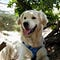Photo by Lilian Joore on Unsplash

# The Wavelet Transform

The Wavelet transform of an image is a hierarchical way to break an image down into different sub-bands. These sub-bands are computed by convolving the image with a wavelet function in either the horizontal or vertical direction and down-sampling the result. For a single level 2d Wavelet transform, we will get 4 subbands: LL, HL, LH, and HH.

• LL: Horizontal and vertical approximate coefficients
• LH: Vertical Approximate, Horizontal Detail
• HL: Vertical Detail, Horizontal Approximate
• HH: Horizontal and Vertical Detail

To further break the image down, we can continue to apply the transform to the LL subband. The lower levels (the coarse scales) of the transform encode high level information about the image whereas higher levels (the fine scales) encode details such as edges. This gives us a hierarchy where low levels are “more important” than higher levels in terms of understanding what is in the image.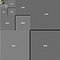A 4-level Daubechies wavelet decomposition of the dog

Notice how the horizontal detail (LH) appear to have vertical streaks. This is because those are where the vertical edges are. Likewise, the vertical detail has horizontal streaks because those are where horizontal edges are. In each sub-band, you can still pick out features which tell you that the image is a dog. At the lowest sub-band (LL1), the image is essentially a small dog!

(Side Note: The black bars are because sub-bands can have different dimensions, so zero-coefficients are appended to make the dimensions equal when showing the decomposition)

This hierarchical nature of wavelets makes them very useful for embedded encodings because their very structure is embedded. If I were to zero-out on of the higher level sub-bands, I could still recover most of the image since I would only lose out on some fine details.

The `pywavelet` package makes computing and visualizing wavelet transforms incredibly easy. Let’s start building our encoder and decoder by defining two classes `ZeroTreeEncoder` and `ZeroTreeDecoder`. These will be responsible for implementing the EZW algorithm and will implement the bulk of our compression.

The encoder is easy: we just take an image (with a single channel), our wavelet, and call the `wavedec2` function to get the wavelet coefficients. They are in the format `[LL1, (LH1, HL1, HH1), (LH2, HL2, HH2),...]`. The function will compute as many levels as possible.

For decoding, we won’t know the actual image, only its size. So we use a 0-image to get an array of coefficients (all 0) which we can build from. At the end of the decoding, we will call the `getImage` function to recover the actual image from the coefficients.

# Quantization

Now that we have our wavelets, we just need to quantize them. To keep things simple, we’ll apply uniform quantization. This means each coefficient is scaled by the same amount (by one in our case). We do this by ceiling the negative coefficients and flooring the positive coefficients (effectively chopping off the decimal places).

We put all the coefficients into a single array using `pywt.coeffs_to_array`, quantize them, and bring them back to the hierarchical format with `pywt.array_to_coeffs`. See the documentation of these functions to clarify what they are doing.

We don’t need to modify the decoder because we only lost decimal places from the quantization, so there is no need to do anything special when unquantizing.

# The EZW Algorithm — Encoding

At this point, we’ve completed the transform and quantization parts of our compression. The only thing left is the entropy coding. This is where we use as few bits as possible to encode our wavelet coefficients. The key to doing this is determining where the significant coefficients are and encoding those first. Doing this lies on two assumptions:

1. Significant coefficients are those with large positive or negative values.
2. If a coefficient at a coarse scale is insignificant, then a coefficient at a finer scale is also insignificant.

In general, the second assumption does not always hold true, but when it is true, we don’t need to encode a potentially large chunk of the coefficients because we know they are insignificant.

To make this more concrete, we say a wavelet coefficient x is insignificant with respect to a threshold T if |x|<T.

A parent is a coefficient at a coarse scale while a child is a coefficient in the next finer scale in the same location as the parent. The descendants of a coefficient are all coefficients at a finer scale for a given parent. Essentially, the wavelet coefficients form a tree where each coefficient has 4 children (except for coefficients in LL1, the coarsest level, which only have 3 children).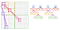An illustration of a few parent-child dependencies

In the illustration above, each large colored square is a sub-band and each black square is a single coefficient. Use the illustration to make sure you understand how the locations of parents and children relate to each other. Notice that each child is in the same type of sub-band as its parent (i.e a parent in LH will have children in LH).

EZW relies on these parent-child dependencies, so to help us write the algorithm, lets build these trees from our wavelet coefficients. We’ll do this in a `CoefficientTree` class.

A `CoefficientTree` knows its value, the wavelet level it is in, which sub-band it belongs to (the quadrant), and its location in the sub-band. We define the `build_trees` method to take wavelet coefficients and return the Coefficient Trees. `build_trees` uses a recursive helper method which given a level, location, and quadrant computes the coordinates of the children and builds their CoefficientTrees. With each recursive call, the level increases by 1 but the quadrant stays the same. The quadrants map numbers to each sub-band. LH is 0, HL is 1, and HH is 2. The LL quadrant is `None`.

Now that we have this tree data structure, we define a coefficient to be part of a zero-tree if it and all its descendants are insignificant. The coefficient is a zero-tree root if it is not the descendant of a previously found zero-tree root. In other words, once we encode the location of a zero-tree root, we don’t have to encode the location of its descendants because they are assumed to be insignificant. However, because assumption 2 is not always true, we will sometimes find isolated zeros, coefficients which are insignificant but have significant coefficients.

This gives us the first part of the Zero-Tree algorithm: encode the locations of the significant coefficients. To make sure that no child is encoded before the parent and that we skip insignificant coefficients, we can perform a breadth-first search on the Coefficient Trees!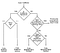Zero-tree flow chart

We’ll follow the above flow chart to perform the dominant pass, using the symbols “P”, “N”, “Z”, “T” for positive, negative, isolated zero, and Zero-tree root.

The symbol for each coefficient is computed in `CoefficientTree#zero_code`. It recurses through the children of the tree and computes their code before computing its own code. Coefficients in the finest sub-bands (i.e the ones with no children) are encoded as zero-tree roots if they are insignificant. With `CoefficientTree#zero_code` doing the encoding, `ZeroTreeEncoder#dominant_pass` is just breadth-first search which ignores children whose parents are zero-tree roots.

Now that we have the locations of significant coefficients, we now need to encode their values. This is done in the subordinate, or secondary, pass. We define our threshold to be the largest power of two less than the maximum absolute coefficient value.

This will guarantee that we have some significant coefficients to begin with. It’s also a good choice because it gives us a creative way to encode the value of the coefficients. As we perform the dominant pass, we will store the significant coefficients in a “secondary list”. Then in the subordinate pass, we will go through the secondary list and encode an additional bit.

To understand this better, consider the following two coefficients: 63 and 47 with a threshold of 32.

The most significant bit (MSB) tells us that both are greater than 32, hence the dominant pass tells us the first bit is 1. During the secondary pass, we need to encode the second MSB, so we should compare the 63–32=31 and 47–32=15 to 16. This gives us 1 and 0 respectively, the second most significant bit!

Putting this into code now, we will slightly modify the `ZeroTreeEncoder#dominant_pass` to return the secondary list and create the `ZeroTreeEnder#secondary_pass` function to perform the subordinate pass.

From here, the rest of the EZW algorithm comes naturally. Divide the threshold by 2 and keep alternating dominant and secondary scans until the threshold is either 0 or there are no more bits to encode. Each time we do a dominant pass, we set the significant coefficients to 0 after they are added to the secondary list so we don’t add them multiple times. This process of encoding the bit-planes of the wavelet transform is known as successive approximation. We use the `bitarray` library to store the bits.

We’ll wrap up the EZW algorithm by making the `ZeroTreeEncoder` class an iterator. That way, we only do as much computation as required, and we can stop after any number of scans are computed.

Each time the iterator is called, we perform either a dominant or subordinate pass and return the result. Notice we save the starting threshold to a variable called `start_thresh`. We’ll need that while decoding!

What makes this process incredibly interesting is that it effectively encodes the bitplanes of the image, and it does so efficiently by ignoring leading zeros. To conceptualize a bitplane, imagine you have a 2D array of numbers (a.k.a an image). You write these numbers in binary and prepend zeros so they all have the same length. You now have a 3D array of 1s and 0s. Each bitplane is a 2D slice of the array.

EZW encodes these bitplanes because a coefficient is only significant if it is larger than the threshold, so it will only be encoded once we reach the threshold which represents the first occurrence of a 1 bit in its binary representation (hence ignoring the leading zeros). After it is on the secondary list, each subordinate pass takes another bit as described before. If you’ve understood this point, then you have understood completely how EZW works.

# The EZW Algorithm —Decoding

Once we’ve finished and understood encoding, decoding becomes a little easier — just do everything in reverse! Remember that our decoder starts out with all coefficients being zero, and we know the start threshold.

Now for the dominant pass, we can fill in significant coefficients with the threshold value because the coefficient is at least as great as the threshold. Given the list produced by the dominant pass, we know which coefficient each symbol corresponds to because we know they were encoded in breadth-first order and no coefficient appears in the dominant list twice.

During the secondary pass, we modify the coefficient value using half the current threshold because the because the bitarray produced by the subordinate pass gives us the next bit of precision.

# Entropy Coding

Now that we’ve implemented EZW, the only thing left to do is the entropy coding. Even though EZW gave us an embedded way to organize the image information, notice that it didn’t actually compress anything! Storing the dominant pass symbols take 8 bits per character if we were to embed them as ASCII. This is clearly sub-optimal.

To keep things simple, we will encode the dominant pass using a prefix-free code. These guarantee we can uniquely decode the symbols from a given sequence of bits. The prefix-free code we will use is based on the fact that we expect there to be a lot of zero-tree roots and few significant coefficients because of the way we progressively lower the threshold. This gives us the following code:

• T: 0
• Z: 10
• P: 110
• N: 111

We don’t need to do anything special with the subordinate pass because it is already expressed in binary.

To keep our function headers the same and abstract away some of the logic of what goes on in the `ZeroTreeEncoder` and `ZeroTreeDecoder` classes, we’ll create a `ZeroTreeScan` class to do the entropy coding and store the bits for us.

We also create a single method `ZeroTreeDecoder#process` to handle the decision of what to do when given a `ZeroTreeScan`.

# Writing the File Format

Now that we’ve done the “compression” part, the last thing we need to do is save it to a file. For the most part, it is straight-forward, but there are a couple of nuances.

Remember, these files need to be completely self-contained. That means all information necessary to reconstruct the image must be either assumed or in the file itself. Because everything looks like 1s and 0s, we also need to somehow mark where pieces of information begin. To do this, we’ll create three markers: Start of Image (SOI), Start of Scan (SOS), and End of Image (EOI). These will be 16 bit “reserved” bytes and are the similar to the ones used by JPEG.

The other thing we need to take care of is the fact that a color image has 3 channels. Our EZW algorithm only works with a single channel image, so we effectively need to run 3 encoders: one for each channel.

We also need to save the image dimensions and the start threshold of each `ZeroTreeEncoder` so our decoder has enough information to start decoding. We’ll put these attributes in the file header between the SOI marker and the first SOS marker.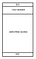Our File Format

We’ll use the `db2` Wavelet from the Daubechies family since it gives empirically good results.

Notice a couple of things:

1. We use `float` instead of `uint8` to represent our images.
2. We encode both dimensions (as well as the start thresholds of each encoder) as 2-bytes each in big-endian format. This means we can encode an image with maximum size of 65536 x 65536
3. We a have a user-specified parameter `max_passes` which controls how many scans we add to the image. This effectively controls the quality of the encoding.

There are a couple of things that I’ve glossed over though. First, we don’t encode the image in RGB space. The reason is that while RGB is the format computers use to display images, each channel is largely redundant. Instead, we transform the image to the YCbCr space.

Y is the illumination channel while Cb and Cr and the blue and red chroma. There is lots of spatial redundancy in the Cb and Cr channels, so we can down-sample them before encoding them to compress the file even more. The transformation from RBG to YCbCr and back is just a linear matrix transformation.

The second thing is that not all channels will have the same number of scans, so we need to check that a scan is not none before writing it, and we are only done when all encoders have no scans.

Finally, we need to write the `ZeroTreeScan#to_file` method in line 43 to write the scans to the file. To do this, we pad the scan to be a multiple of 8 bits and then write it to the file. We pad to 8 bits because our markers are 2 bytes, so we will need to read the image 1 byte at a time while decoding to detect the markers properly. We pad with 0s because 0 is decoded to T by the prefix-free code, so we won’t change any coefficient values.

We also do something called byte-stuffing. Notice that it is possible for our SOI, SOS, and EOI markers to appear in our scans. If this happens, it will be impossible to decode! To avoid this, we search for any `FF` bytes and replace them with `FF00`. That way, while decoding, if we encode an `FF00`, we can replace it with `FF`. This sacrifices a little compression for decodability.

# Reading the File Format

Once we’ve written the file format, decoding it is just doing the reverse. First, we read the file header to get the image dimensions and initialize our decoders. Then we read as many scans as we can, making sure to replace any stuffed bytes with `FF` and stopping only when we find the `EOI` marker. We do this by creating a buffer and filling it by reading the image 1 byte at a time. Once we hit a marker, we split the buffer into the data and the marker and process the data. When no scans remain, we convert back to YCbCr.

# Conclusion

Now that we’ve build our Encoder and Decoder, all we need to do is try it out! Here are a couple of outputs with a different number of `max_passes` for the dog image and the corresponding compression ratio.

Compressing the image to 1/257th its original size still gives us a recognizable dog, and with just 10 more passes, our image is essentially perfect but only 1/6th the size! You might notice that the quality of 10 passes looks a lot like how video streaming platforms look with low network connection: its very likely because of embedded encoding.

Another important thing to notice is that unlike JPEG, we don’t get any block artifacts at low qualities. This is because we took the wavelet transform of the entire image rather than 8x8 blocks like JPEG does.

Debugging this system end-to-end can be difficult, so if you find things aren’t working, remember to test each component separately. If you find the EZW algorithm isn’t working properly, try performing the algorithm by hand on a small example to make sure your code reflects the algorithm. If you find the writing to a file isn’t working properly, double check that bit-padding and byte-stuffing are working and that you can detect the markers.

# Where To Go From Here

There are a lot of ways to improve this algorithm. This article focused on the EZW algorithm, so many of the other pieces of compression are very simplified. This is why our encoder/decoder does not perform quite as well as JPEG.

Here are some places it could be improved:

1. More clever quantization. We can quantize the CbCr channels more heavily (like JPEG does), or we can quantize different levels of the wavelet transform differently.
2. In Shapiro’s paper, he used Adaptive Arithmetic coding on both the dominant and subordinate scans instead of a prefix-free code
3. Write it in C or C++ to make it faster
4. Change the wavelet. We want to concentrate the image’s “energy” into as few coefficients as possible, and some wavelets are better at this than others.
5. Change the quality level from `max_passes` to a bit rate

I hope you found this tutorial useful. You can find all of the code on GitHub. Feel free to leave any questions in the comments!

## Sign up for Top 10 Stories

### By The Startup

Get smarter at building your thing. Subscribe to receive The Startup's top 10 most read stories — delivered straight into your inbox, once a week. Take a look.

By signing up, you will create a Medium account if you don’t already have one. Review our Privacy Policy for more information about our privacy practices.

Check your inbox
Medium sent you an email at to complete your subscription.

Written by

## Anmol Parande

Student of Electrical Engineering and Computer Science at UC Berkeley

## The Startup

Get smarter at building your thing. Follow to join The Startup’s +8 million monthly readers & +786K followers.

Written by

## Anmol Parande

Student of Electrical Engineering and Computer Science at UC Berkeley## The Startup

Get smarter at building your thing. Follow to join The Startup’s +8 million monthly readers & +786K followers.

## More From Medium

Medium is an open platform where 170 million readers come to find insightful and dynamic thinking. Here, expert and undiscovered voices alike dive into the heart of any topic and bring new ideas to the surface. Learn more

Follow the writers, publications, and topics that matter to you, and you’ll see them on your homepage and in your inbox. Explore

If you have a story to tell, knowledge to share, or a perspective to offer — welcome home. It’s easy and free to post your thinking on any topic. Write on Medium